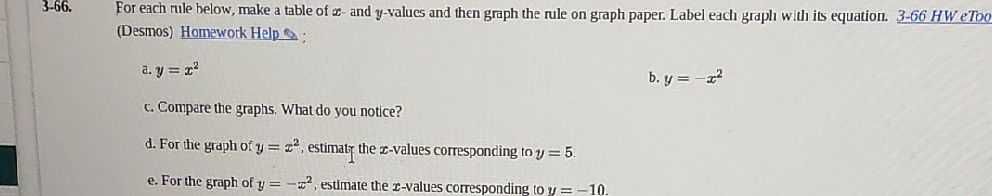### ¿Todavía tienes preguntas de matemáticas?

Pregunte a nuestros tutores expertos
Algebra
Pregunta3.66. For each rule below, make a table of $$x$$ -and $$y$$ -values and then graph the rule on graph paper. Label each graplı with its equation. $$3$$ -66 HW eToo (Desmos) Homewotk Help

a. $$y = x ^ { 2 }$$

c. Compare the graphs. What do you notice?

d. For the graph of $$y = x ^ { 2 }$$ , estimatr the $$x$$ -values corresponding to $$y = 5$$ .

e. For the graph of $$y = - x ^ { 2 }$$ , estimate the $$x$$ -values corresponding to $$y = - 10$$ .

d.$$\pm \sqrt{5}$$